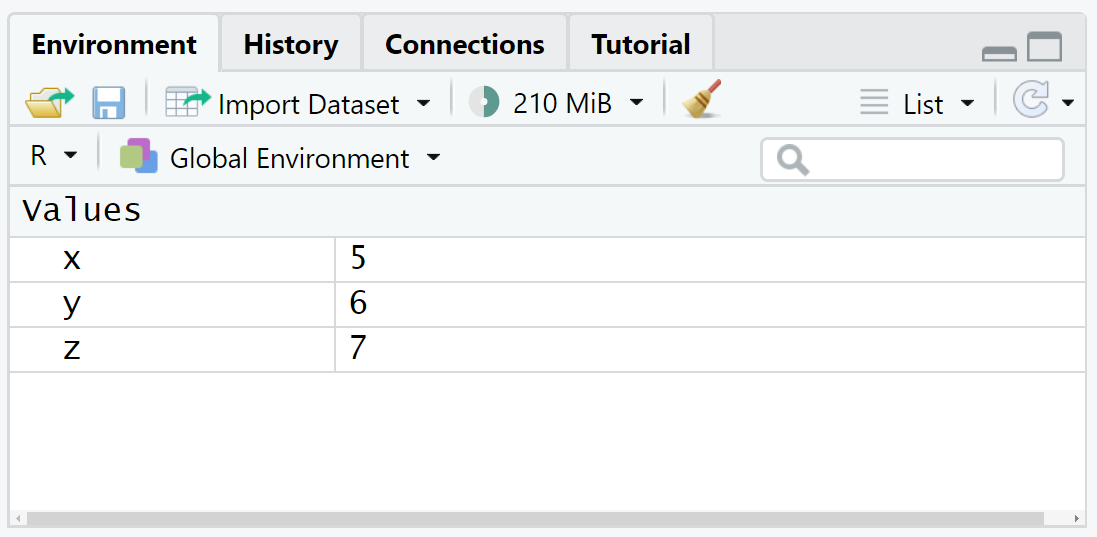# What’s the Difference between rm() & rm(list=ls()) in R? (Example Code)

In this tutorial, I’ll demonstrate how to apply the rm function to clear a workspace in the R programming language.

The content of the tutorial is structured as follows:

Let’s jump right to the example.

## Example Data

Let’s first define some example data in R:

```x <- 5                     # Create some data objects
y <- 6
z <- 7```

After running the previous R code, our Global Environment in RStudio looks as follows:As you can see, our workspace contains the three data objects x, y, and z.

## Example 1: Apply rm()

The code below demonstrates what happens when we apply the rm function without any further specifications.

Let’s just do this:

`rm()                       # Try to clear workspace`

Have a look at the Global Environment of your RStudio session after running the previous syntax. As you can see, the workspace has not changed at all.

The reason for this is that we have not specified any data objects within the rm function that we want to remove.

Hence, the rm function has not deleted any data objects or variables.

## Example 2: Apply rm(list = ls())

Let’s see what happens when we run the R code rm(list = ls()):

`rm(list = ls())            # Properly clear workspace`As you can see, we have cleared all data objects from our currently used workspace.

The reason why it has worked this time is that the ls() function returns a list of all data object names in our workspace.

Hence, R removes everything from the environment after running the previous code.

## Video & Further Resources

In case you need further explanations on the topics of this tutorial, I recommend watching the following video on my YouTube channel. In the video, I’m explaining the content of this article:

In addition, you might read the other tutorials on this website. A selection of articles on related topics such as ggplot2 and time objects is listed below.

In this R tutorial you have learned how to use the rm function to clear a workspace. Don’t hesitate to let me know in the comments below, in case you have additional questions.

Subscribe to the Statistics Globe Newsletter